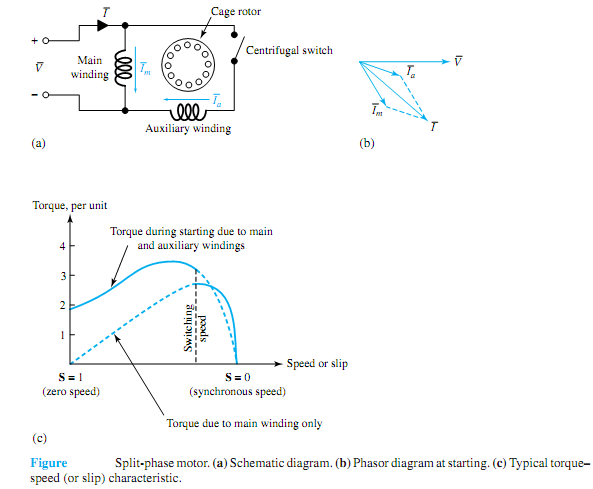## Split-phase or resistance-split-phasemotor, Electrical Engineering

Assignment Help:

Q. Split-phase or resistance-split-phasemotor?

Split-phase or resistance-split-phasemotors: Split-phasemotors have two statorwindings (amainwinding and an auxiliarywinding)with their axes displaced 90 electrical degrees in space. As represented schematically in Figure (a), the auxiliary winding in this type of motor has a higher resistance-to-reactance ratio than the main winding. The two currents are thus out of phase, as indicated in the phasor diagram of Figure (b). The motor is equivalent to an unbalanced two-phase motor. The rotating stator field produced by the unbalanced two-phase winding currents causes the motor to start. The auxiliary winding is disconnected by a centrifugal switch or relaywhen themotor comes up to about 75%of the synchronous speed.The torque-speed characteristic of the split-phase motor is of the form shown in Figure (c). A split-phase motor can develop a higher starting torque if a series resistance is inserted in the starting auxiliary winding. A similar effect can be obtained by inserting a series inductive reactance in the main winding; this additional reactance is short-circuited when the motor builds up speed.#### Estimate the bandwidth by using carsons rule, Let a message signal m(t) hav...

Let a message signal m(t) have a bandwidth of 10 kHz and a peak magnitude of 1 V. Estimate the bandwidth, by using Carson's rule, of the signal u(t) obtained when m(t) modulates a

#### 3-phase 180 degree mode vsi , 3-phase 180 0 mode VSI In 1800 mode VSI...

3-phase 180 0 mode VSI In 1800 mode VSI  each thyristor  conduct  for 1800 and two  thyristor  are fired  at 600 interval. For  example  if thyristor  T1  is fired at 00 then

#### For zero flag , For zero flag  CZ ( Call  on Zero )  CNZ ( Call on no...

For zero flag  CZ ( Call  on Zero )  CNZ ( Call on no zero ) Instructions CZ call the  subroutine  from the  specified  memory location if zero flag is set  (Z=1). The i

#### Finite state machine, the tutorial assignment is part of my thesis work and...

the tutorial assignment is part of my thesis work and i need help in it please

#### Unit to measure the electric charge q, Unit to measure the electric charge ...

Unit to measure the electric charge Q?? The unit used to measure the quantity of electrical charge Q is called the coulomb C

#### Interfaced 2k x 8 eprom with multiple input nand gate, Interfaced 2k X 8 (i...

Interfaced 2k X 8 (i.e 2716) EPROM using multiple input NAND gate decoder for memory locations FF800H-FFFFFH. Simple NAND gate Decoder:  While the 2k x 8 EPROM is used so addre

#### Find the phasor values with peak magnitude, Q. For the circuit shown in Fig...

Q. For the circuit shown in Figure, find the phasor values (with peak magnitudes) of ¯I, ¯V R , ¯V L , and ¯V C by using PSpice.

#### Find the power factor and current of the machine, Two identical three-phase...

Two identical three-phase, 33-kV, wye connected, synchronous generators operating in parallel share equally a total load of 12 MW at 0.8 lagging power factor. The synchronous react

#### Frequency response of your amplifier, a) What is meant by frequency respons...

a) What is meant by frequency response and how would you check the performance of your amplifier? b) Calculate the Voltage Gain of your amplifier at a range of frequencies suffic

#### Find the fourier-series expansion of the pulse train, The rectangular pulse...

The rectangular pulse train of Figure (a) consists of pulses of height A and duration D. Such pulse trains are employed for timing purposes and to represent digital information. Fo